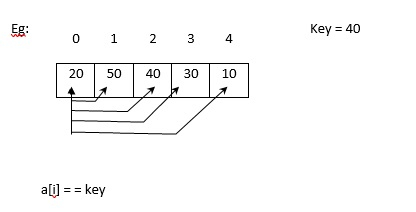# How to find minimum element in an array using linear search in C language?

C programming language provides two types of searching techniques. They are as follows −

• Linear search
• Binary search

## Linear Search

• Searching for the key element is done in a linear fashion.
• It is the simplest searching technique.
• It does not expect the list to be sorted.
• Limitation − It consumes more time and reduce the power of system.
Input (i/p): Unsorted list of elements, key.
Output (o/p):
• Success – If key is found.
• Unsuccessful – Otherwise.## Example1

Following is the C program to find minimum element in an array by using linear search −

Live Demo

#include<stdio.h>
int main (){
int a, n, i, key, flag = 0;
printf("enter the no: of elements");
scanf ("%d",&n);
printf("enter the elements:");
for (i=0; i<n; i++)
scanf( "%d", &a[i]);
printf("enter a key element:");
scanf ("%d", &key);
for (i=0; i<n; i++){
if (a[i] == key){
flag = 1;
break;
}
}
if (flag == 1)
printf("search is successful:");
else
printf("search is unsuccessfull:");
return 0;
}

## Output

When the above program is executed, it produces the following result −

enter the no: of elements 5
enter the elements:
12
34
56
78
89
enter a key element:56
search is successful:

## Example2

Given below is another program to find the minimum element in array by using liner search −

Live Demo

#include <stdio.h>
int min_ele(int numbers[], int n){
int min = numbers;
int i;
for (i = 1; i <= n; i++){
if (min > numbers[i])
min = numbers[i];
}
return min;
}
int main(){
int n;
printf("Enter no: of elements in an array: ");
scanf("%d",&n);
int numbers[n];
int i;
int min ;
printf("Enter %d numbers : ", n);
printf("");
for (i = 0; i < n; i++){
scanf("%d", &numbers[i]);
}
min = min_ele(numbers,n);
printf("\In an array the minimum number is: %d", min);
return 0;
}

## Output

When the above program is executed, it produces the following result −

Enter no: of elements in an array: 5
Enter 5 numbers:
23
56
78
9
20
In an array the minimum number is: 9